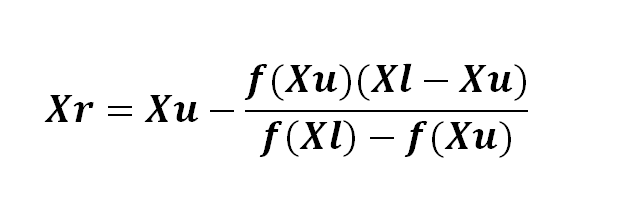#### How to calculate false position?

To understand how to calculate false position in Excel, we are going to first introduce you to the whole method.

That’s an “algorithm for finding roots which retains that prior estimate for which the function value has the opposite sign from the function value at the current best estimate of the root.”

## How to calculate?

The example that we are using consists of five attributes. They are all placed well in a table.

We have two values that are already set, xl and xu. For the sake of this article, their values are 0 and 2.

We are first going to calculate f(xl) and f(xu).

We are going to use this formula:

=EXP(-x^2)-x-10

Replace all X’s with the coordinates of xl’s cell. In our case, the result we got was -9. We are going to do the same thing now with f(xu), and by doing that we got 42.5982.

Now we are left with xr. We are going to calculate it with this formula:This may seem like a lot of brackets, but that’s because you have to replace all the X’s with the coordinates that match them.

The result we got is 0.34885. That’s how yours should’ve turned out if you followed the same example.

And the last thing that we have to calculate is f(xr). We are going to use the same formula that we used for calculating f(xl) and f(xu), and the result is -9.2194.

To fill up the other rows, are you going to start by creating a new xl and xu.

For a new xl use this formula:

=if(f(xl)*f(xr)<0,xl,xr)

For a new xu use this formula:

=if(f(xl)*f(xr)<0,xr,xu)

Copy and paste the rest and if everything works, you did it. You used the false position method.

## Conclusion

Calculating false position solves all non-linear equations quickly through numerical methods. It will definitely help you out and ease the whole process with the help of just a few formulas.